# The NLMIXED Procedure

### Hierarchical Model Specification

PROC NLMIXED supports multiple RANDOM statements to accommodate nested multilevel nonlinear mixed models, starting with SAS/STAT 13.2. If you use multiple RANDOM statements, PROC NLMIXED assumes that the SUBJECT= variable from each RANDOM statement forms a containment hierarchy. In the containment hierarchy, each SUBJECT= variable is contained by another SUBJECT= variable, and the SUBJECT= variable that is contained by all SUBJECT= variables is considered "the" SUBJECT= variable. For example, consider the following three-level nested model that has three SUBJECT= variables:

 A B C 1 1 1 1 1 2 1 2 1 1 2 2 2 1 1 2 1 2 2 1 3 2 2 1 2 2 2

Suppose you specify a three-level nested model by using the following three RANDOM statements:

random r11 ~ normal(0,sd1) subject = A;
random r21 ~ normal(0,sd2) subject = B(A);
random r31 ~ normal(0,sd3) subject = C(A*B);


Then PROC NLMIXED assumes the containment hierarchy as follows. The first-level hierarchy is defined using "the" SUBJECT= variable A. Similarly, the second-level hierarchy is defined using SUBJECT= variable B, which is nested within first level. Finally, SUBJECT= variable C defines the third-level hierarchy that is nested within both the first and second levels. In short, the SUBJECT= variable A is "the" SUBJECT= variable. B is contained in A, and C is contained in both A and B. In this example, there are two first-level subjects that are determined using "the" SUBJECT= variable A.

Based on the preceding hierarchy specification, PROC NLMIXED’s indexing of nested subjects for each first-level subject can be visualized as follows: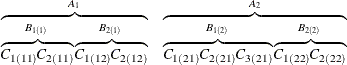The ith subject from the first level is denoted as A, the second-level nested subjects are denoted as B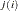, and the third-level nested subjects are denoted as C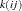.

You can specify any nested structure by using the SUBJECT= syntax in PROC NLMIXED. For example, using the following three RANDOM statements, PROC NLMIXED fits a different model:

random r11 ~ normal(0,sd1) subject = C;
random r21 ~ normal(0,sd2) subject = B(C);
random r31 ~ normal(0,sd3) subject = A(C*B);


In this case, PROC NLMIXED processes the subjects by using SUBJECT= variable C as "the" SUBJECT= variable, and the containment hierarchy is changed as follows:

 A B C 1 1 1 1 1 2 1 2 1 1 2 2 2 1 1 2 1 2 2 1 3 2 2 1 2 2 2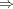C B A 1 1 1 1 1 2 1 2 1 1 2 2 2 1 1 2 1 2 2 2 1 2 2 2 3 1 2

Again, PROC NLMIXED’s indexing of the nested subjects in this containment hierarchy can be visualized as follows: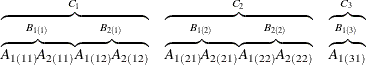Here, PROC NLMIXED assumes that C is "the" SUBJECT= variable. B is contained in C, and A is contained in C and B. In this case, there are three first-level subjects that are determined using "the" SUBJECT= variable C.

As explained before, in this case, the ith subject from the first level is denoted as C, the second-level nested subjects are denoted as Band for third level, the nested subjects are denoted as A.

Note that the containment hierarchy could potentially create more subjects than the unique number of subjects. For example, consider the following table, in which A is "the" SUBJECT= variable and B is nested within the subject A:

 A B a 1 a 2 b 1 b 2 c 1 c 2

Even though the SUBJECT = B variable has only two unique subjects (1 and 2), when the containment hierarchy that is specified along with B is nested within A, PROC NLMIXED creates six nested B subjects. These nested subjects can be denoted as 1(a), 2(a), 1(b), 2(b), 1(c), and 2(c).

PROC NLMIXED does not support noncontainment hierarchy (or non-nested) models. For example, the following statements are not supported, because subject C is not nested within B and A:

random r11 ~ normal(0,sd1) subject = A;
random r21 ~ normal(0,sd2) subject = B(A);
random r31 ~ normal(0,sd3) subject = C;